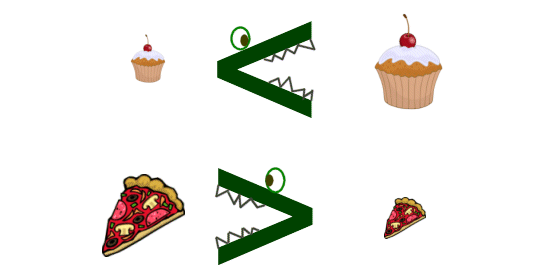# Kids Math

## Inequalities

In most math problems you are trying to find the exact answer. We use the equal sign "=" to say that two things are the same. However, sometimes we just want to show that something is bigger or smaller than something else. Or maybe we just want to say that two things are not equal. These cases are called inequalities.

Special Signs

There are special signs used with inequalities to indicate which side is bigger, which side is smaller, or that the two sides aren't equal.

Here are the five major inequality signs:

 < ≤ > ≥ ≠ less than less than or equal to greater than greater than or equal to not equal

Greater Than or Less Than

When you want to say that one thing is bigger than another, you use the greater than or less than signs. You put the wide part of the sign towards the greater side and the small part, or point, towards the smaller side.

Examples:

8 > 3
4 < 9
0 < 12

You could also use variables like this:

a + b < 17
22 > y
(x + y) x 8 < z

If you need to remember which way the greater than or less than sign should point, you can remember it this way. Think of the sign as an alligator's mouth. The alligator wants to eat the larger side. Like this:When we want to say something is greater than or equal to something else, we add in an equal sign. This symbol looks like this: . As you can see it's sort of a combination of the > sign plus the = sign.

We use the opposite type of sign when we want to indicate less than or equal to, like this: .

Example Problems:

1) The number X could be 3 or any number greater than 3. You could write this as:

X ≥ 3

2) The number Y could be 2 or any number less than 2. You could write this as:

Y ≤ 2

3) Billy had 6 candy bars. Amy ate some of his candy bars. How many candy bars does Billy have now?

# candy bars < 6

4) Jacob had 11 math problems for homework. We know he got 4 problems correct, but we don't know the results of the other problems. How many did Jacob get correct?

Multiple Inequalities

Sometimes you can use more than of these signs in the same expression in order to indicate a range. For example, if you had between 3 and 9 apples you would write:

3 < apples < 9

If you had at least 12 marbles and as many has 20 marbles:

12 ≤ marbles ≤ 20

Kids Math Subjects

Back to Kids Math

Back to Kids Study